Author: Oscar Cronquist Article last updated on April 02, 2020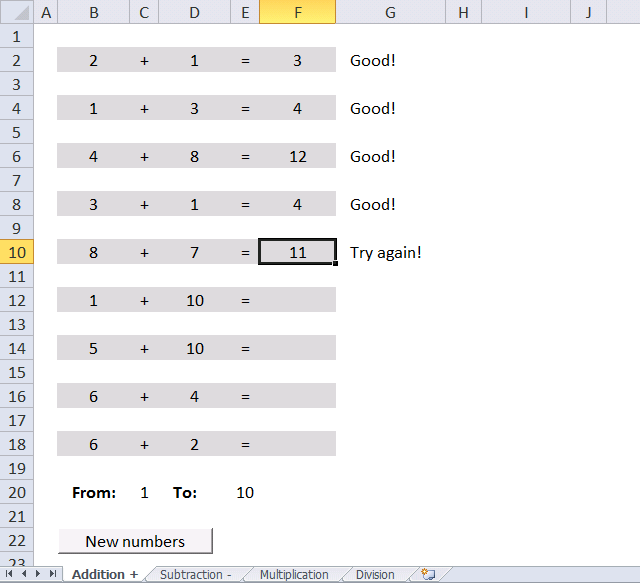This article demonstrates a workbook that allows children to practice basic mathematics or more specifically arithmetic calculations. The image above shows a worksheet named "Addition", it allows the user to practice adding numbers.

There are four worksheets in the workbook and each worksheet shows numbers in columns B and D. The user then types the result in column F and Excel responds in column G if the answer was right or wrong.

Row 20 lets you control the numerical range that is used, for example, a range between 5 and 10 generates random whole numbers between 5 and 10  in column s B and D. This allows you to control the level of calculation, older children can use more difficult numbers to create a better challenge.

Row 22 contains a button that is linked to a macro named "Newnumbers()", press with left mouse button on the button to create new numbers in columns B and D.

The workbook contains four Excel worksheets named "Addition", "Subtraction", "Multiplication" and "Division". Press with mouse on one of the tabs below the cell grid to change the worksheet, shown in the image above.

Formula in cell B2:

=RandomBetween(\$C\$20,\$E\$20)

This formula uses a User Defined Function that I created. It has two arguments, the lower bound and the upper bound specified in cell C20 and E20.

The VBA code for User Defined Function RandomBetween is shown below.

```'Name UDF and dimension argument variables and declare data types
Function RandomBetween(Low As Long, High As Long)

'Initialize random-number generator
Randomize

'Create a whole random number based on arguments High and Low and return it to the cell in the worksheet
RandomBetween = Int(Rnd * (High - Low + 1)) + Low
End Function
```

Formula in cell G2:

=IF(F2="","",IF(F2=(B2+D2), "Good!", "Try again!"))

The formula in cell G2 has two nested IF functions. The first IF function checks if cell F2 is empty and returns nothing if this is true. Another IF function is rund if this is false, it adds the numbers in cell B2 and D2 and checks if it is equal to the value in cell F2.

If they are equal then text string "Good!" is returned, if not equal text string "Try again" is returned.

The following macro clears cell range F2:F18 in all worksheets in the active workbook. This macro is used in all four worksheets and linked to a button in each worksheet.

```'Name macro
Sub Newnumbers()

'Dimension variables and declare data types
Dim sht As Worksheet

'Iterate through each worksheet in active workbook using variable sht as worksheet
For Each sht In ActiveWorkbook.Worksheets

'Clear cell range F2:F18 based on variable sht
sht.Range("F2:F18") = ""

'Continue with next worksheet
Next sht

'Forces a full calculation of the data in all open workbooks.
Application.CalculateFull

End Sub
```

### Subtraction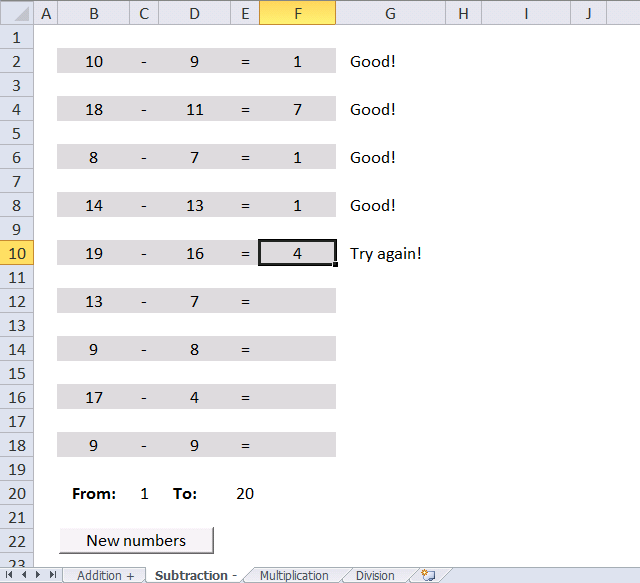Worksheet "Subtraction" lets the user train their subtraction skills. The result is always 0 (zero) or higher. The same User Defined function is used in this worksheet as in worksheet "Addition", however, the second argument is different in column D.

Formula in cell B2:

=RandomBetween(\$C\$20,\$E\$20)

Formula in cell D2:

=RandomBetween(\$C\$20,B2)

If you want to allow negative results change the formula in column D to:

=RandomBetween(\$C\$20,\$E\$20)

Formula in cell G2:

=IF(F2="", "", IF(F2=(B2-D2), "Good!", "Try again!"))

The formula in cell G2 has two nested IF functions. The first IF function checks if cell F2 is empty and returns nothing if this is true. Another IF function is rund if this is false, it subtracts the number in cell B2 with cell D2 and checks if it is equal to the value in cell F2.

If they are equal then text string "Good!" is returned, if not equal text string "Try again" is returned.

### Multiplication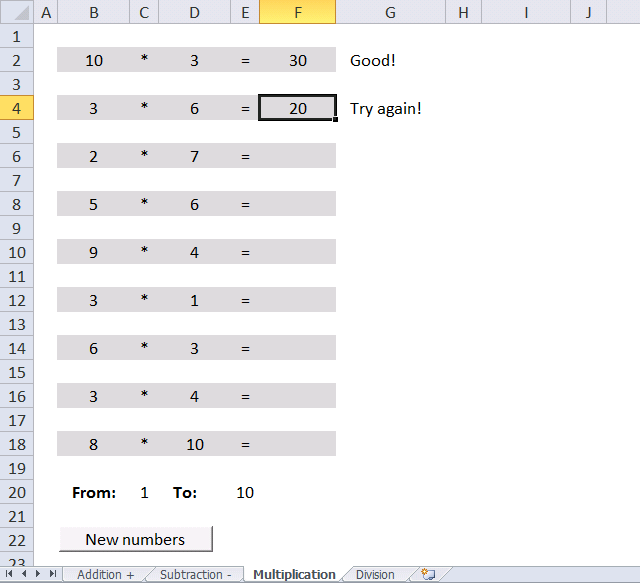Formula in cell B2:

=RandomBetween(\$C\$20,\$E\$20)

Formula in cell B2:

=RandomBetween(\$C\$20,\$E\$20)

Formula in cell G2:

=IF(F2="", "", IF(F2=(B2*D2), "Good!", "Try again!"))

The formula in cell G2 has two nested IF functions. The first IF function checks if cell F2 is empty and returns nothing if this is true. Another IF function is rund if this is false, it multiplies the number in cell B2 with cell D2 and checks if it is equal to the value in cell F2.

If they are equal then text string "Good!" is returned, if not equal text string "Try again" is returned.

### Division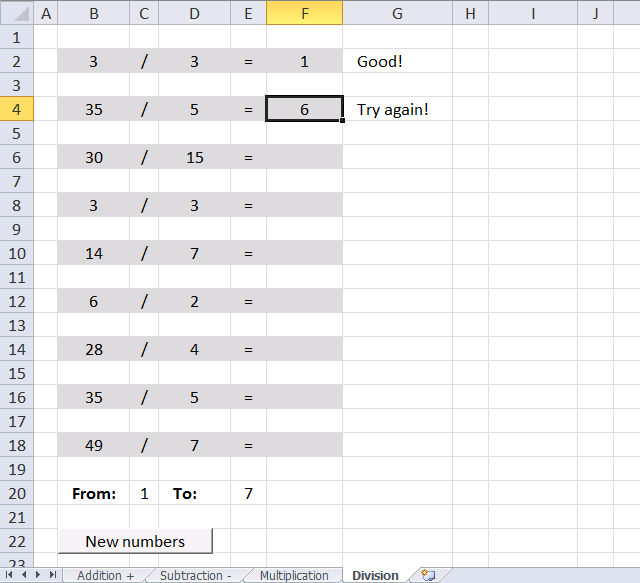This worksheet contains math problems that lets you train divison. Dividend / Divisor = Quotient

This worksheet requires a different User Defined Function to avoid remainders. The number left over is called a remainder.

Formula in cell B2:

=RandomBetweenDivison(\$C\$20,\$E\$20)

Formula in cell D2:

=RandomBetweenDivison(\$C\$20,\$E\$20,B2)
```'Name user defined function and dimension parameters and their data types. Paramter Num is optional meaning it isn't required.
Function RandomBetweenDivison(Low As Long, High As Long, Optional Num As Variant)

'Dimension variables and declare data types
Dim i As Long, str() As Long

'The ReDim statement resizes a dynamic array that has already been declared
ReDim str(0)

'Initialize random-number generator
Randomize

'Check Optional variable Num is missing
If IsMissing(Num) Then

'Create random whole numbers based on parameters High and Low
RandomBetweenDivison = (Int(Rnd * (High - Low + 1)) + Low) * (Int(Rnd * (High - Low + 1)) + Low)
Else
'Iterate from number stored in parameter Num to 1 based on -1 increments
For i = Num To 1 Step -1

'Check if paramater Num divided by variable i is a whole number
If Num / i = Int(Num / i) Then

'Save number in variable i to array variable str
str(UBound(str)) = i

'Add a new container to array variable str
ReDim Preserve str(UBound(str) + 1)
End If
Next i

'Delete last container in array variable str
ReDim Preserve str(UBound(str) - 1)

'Save random whole number based on the number of containers in array variable str to variable i
i = Int(Rnd * (UBound(str)))

'Return value stored in array variable str based on variable i
RandomBetweenDivison = str(i)
End If
End Function
```

### Where to put VBA code?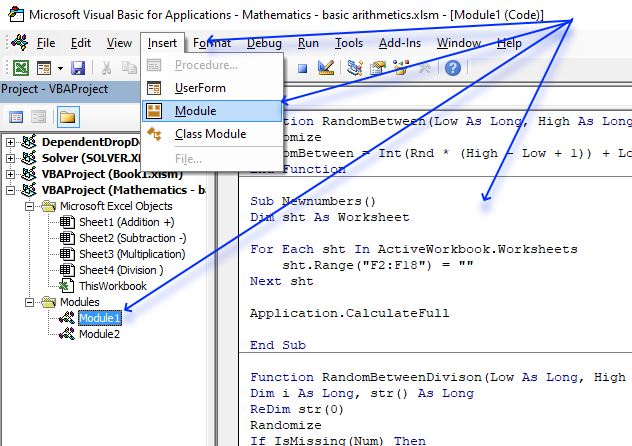1. Copy above VBA code.
2. Press shortcut keys Alt + F11 to open the Visual Basic Editor.
3. Press with mouse on "Insert" on the menu, see image above.
4. Press with mouse on "Module".
5. Paste VBA code to module window.